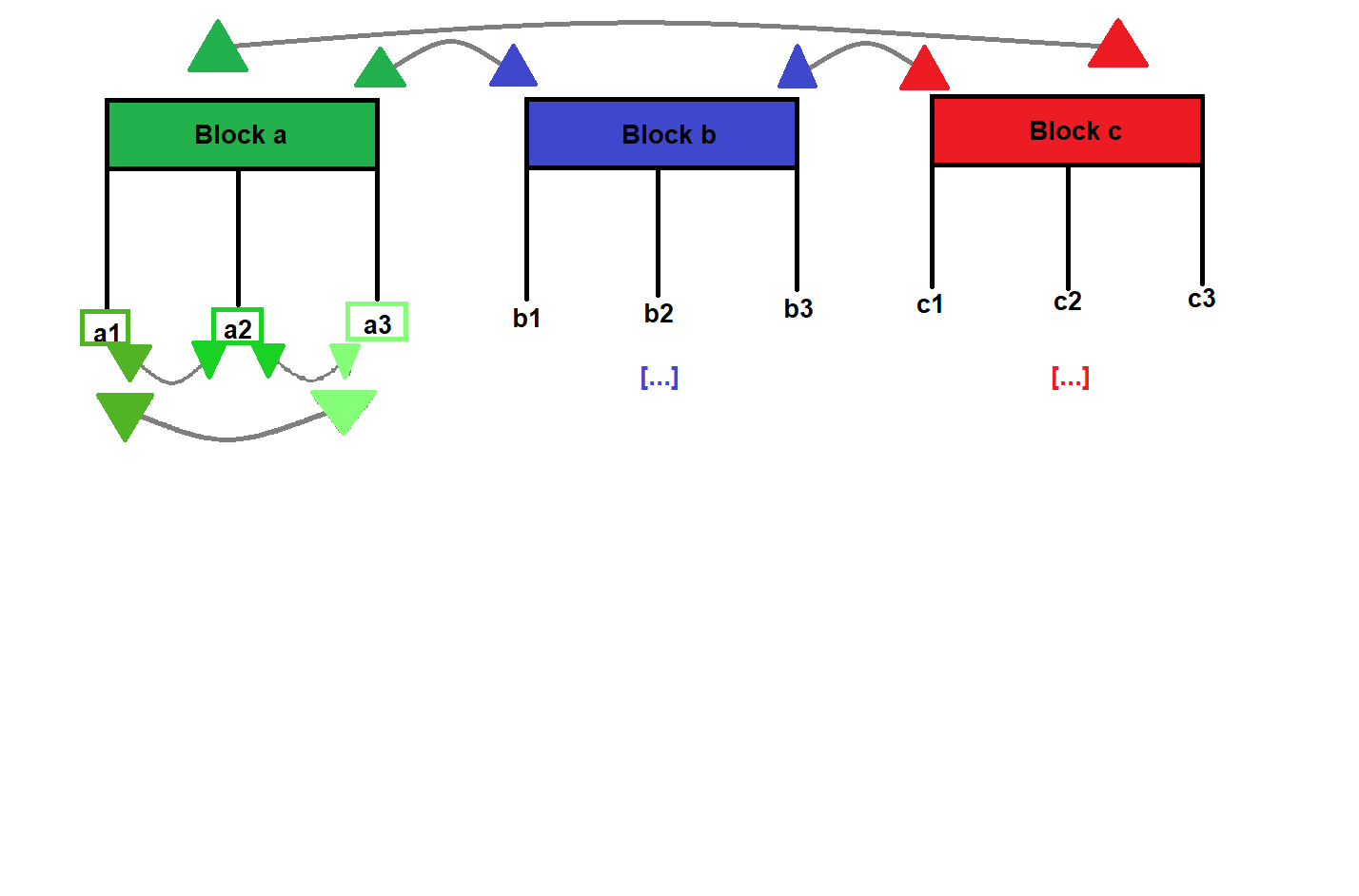## Randomization of blocks and "Sub-Blocks".

##### Randomization of blocks and "Sub-Blocks".
 Author Message DannyPilgrimGroup: Forum Members Posts: 26, Visits: 117 Hello everyone,I hope you understand my question.I have three separate blocks which contain three Sub-blocks each:Block_a- a1 = 96 Trials- a2 = 96 Trials- a3 = 96 TrialsBlock_b- b1 = 96 Trials- b2 = 96 Trials- b3 = 96 TrialsBlock_c- c1 = 96 Trials- c2 = 96 Trials- c3 = 96 TrialsI'd like to randomize the big blocks (Block_a, Block_b, Block_c) and those inside of them as well. The picture visualizes what I mean.On which layer do I have to define this? On the or layer?Would it be something like that in the layer:/ preinstructions = (htmlpage.Übung2)/ trials = noreplace=[1-96 =noreplace(...)]/ trials = [1-96 =noreplace(...)]/ trials = [1-96 =noreplace(...)](The trials themselves are randomized in each Sub-Block with =noreplace)Or do I have to define all Sub-Blocks and arrange them within the layer?I know how to randomize blocks in the but right now I cannot define it how I need it.Best Regards,DannyDannyPilgrimGroup: Forum Members Posts: 26, Visits: 117Daveposted Last Month ANSWERGroup: Administrators Posts: 11K, Visits: 68K +x DannyPilgrim - 4/28/2021You have to do something like this:/ a_blockcount = 0/ b_blockcount = 0/ c_blockcount = 0/ blocks = [1-3 = noreplace(block.a, block.b, block.c)]/ branch = [    //select a random sub-block    list.a_sub.nextvalue;]// three "sub-blocks"/ items = (block.a1, block.a2, block.a3)/ onblockbegin = [    values.a_blockcount += 1;]/ trials = [1=mytrial]/ branch = [    // if we stil have further sub-blocks to run, select the next one at random    if (values.a_blockcount < 3) {        list.a_sub.nextvalue;    }]/ onblockbegin = [    values.a_blockcount += 1;]/ trials = [1=mytrial]/ branch = [    // if we stil have further sub-blocks to run, select the next one at random    if (values.a_blockcount < 3) {        list.a_sub.nextvalue;    }]/ onblockbegin = [    values.a_blockcount += 1;]/ trials = [1=mytrial]/ branch = [    // if we stil have further sub-blocks to run, select the next one at random    if (values.a_blockcount < 3) {        list.a_sub.nextvalue;    }]/ branch = [    //select a random sub-block    list.b_sub.nextvalue;]// three "sub-blocks"/ items = (block.b1, block.b2, block.b3)/ onblockbegin = [    values.b_blockcount += 1;]/ trials = [1=mytrial]/ branch = [    // if we stil have further sub-blocks to run, select the next one at random    if (values.b_blockcount < 3) {        list.b_sub.nextvalue;    }]/ onblockbegin = [    values.b_blockcount += 1;]/ trials = [1=mytrial]/ branch = [    // if we stil have further sub-blocks to run, select the next one at random    if (values.b_blockcount < 3) {        list.b_sub.nextvalue;    }]/ onblockbegin = [    values.b_blockcount += 1;]/ trials = [1=mytrial]/ branch = [    // if we stil have further sub-blocks to run, select the next one at random    if (values.b_blockcount < 3) {        list.b_sub.nextvalue;    }]/ branch = [    //select a random sub-block    list.c_sub.nextvalue;]// three "sub-blocks"/ items = (block.c1, block.c2, block.c3)/ onblockbegin = [    values.c_blockcount += 1;]/ trials = [1=mytrial]/ branch = [    // if we stil have further sub-blocks to run, select the next one at random    if (values.c_blockcount < 3) {        list.c_sub.nextvalue;    }]/ onblockbegin = [    values.c_blockcount += 1;]/ trials = [1=mytrial]/ branch = [    // if we stil have further sub-blocks to run, select the next one at random    if (values.c_blockcount < 3) {        list.c_sub.nextvalue;    }]/ onblockbegin = [    values.c_blockcount += 1;]/ trials = [1=mytrial]/ branch = [    // if we stil have further sub-blocks to run, select the next one at random    if (values.c_blockcount < 3) {        list.c_sub.nextvalue;    }]/ stimulusframes = [1=mytext]/ validresponse = (57)/ items = ("This is block <%script.currentblock%>.") DannyPilgrimGroup: Forum Members Posts: 26, Visits: 117 +x Dave - 4/28/2021+x DannyPilgrim - 4/28/2021You have to do something like this:/ a_blockcount = 0/ b_blockcount = 0/ c_blockcount = 0/ blocks = [1-3 = noreplace(block.a, block.b, block.c)]/ branch = [    //select a random sub-block    list.a_sub.nextvalue;]// three "sub-blocks"/ items = (block.a1, block.a2, block.a3)/ onblockbegin = [    values.a_blockcount += 1;]/ trials = [1=mytrial]/ branch = [    // if we stil have further sub-blocks to run, select the next one at random    if (values.a_blockcount < 3) {        list.a_sub.nextvalue;    }]/ onblockbegin = [    values.a_blockcount += 1;]/ trials = [1=mytrial]/ branch = [    // if we stil have further sub-blocks to run, select the next one at random    if (values.a_blockcount < 3) {        list.a_sub.nextvalue;    }]/ onblockbegin = [    values.a_blockcount += 1;]/ trials = [1=mytrial]/ branch = [    // if we stil have further sub-blocks to run, select the next one at random    if (values.a_blockcount < 3) {        list.a_sub.nextvalue;    }]/ branch = [    //select a random sub-block    list.b_sub.nextvalue;]// three "sub-blocks"/ items = (block.b1, block.b2, block.b3)/ onblockbegin = [    values.b_blockcount += 1;]/ trials = [1=mytrial]/ branch = [    // if we stil have further sub-blocks to run, select the next one at random    if (values.b_blockcount < 3) {        list.b_sub.nextvalue;    }]/ onblockbegin = [    values.b_blockcount += 1;]/ trials = [1=mytrial]/ branch = [    // if we stil have further sub-blocks to run, select the next one at random    if (values.b_blockcount < 3) {        list.b_sub.nextvalue;    }]/ onblockbegin = [    values.b_blockcount += 1;]/ trials = [1=mytrial]/ branch = [    // if we stil have further sub-blocks to run, select the next one at random    if (values.b_blockcount < 3) {        list.b_sub.nextvalue;    }]/ branch = [    //select a random sub-block    list.c_sub.nextvalue;]// three "sub-blocks"/ items = (block.c1, block.c2, block.c3)/ onblockbegin = [    values.c_blockcount += 1;]/ trials = [1=mytrial]/ branch = [    // if we stil have further sub-blocks to run, select the next one at random    if (values.c_blockcount < 3) {        list.c_sub.nextvalue;    }]/ onblockbegin = [    values.c_blockcount += 1;]/ trials = [1=mytrial]/ branch = [    // if we stil have further sub-blocks to run, select the next one at random    if (values.c_blockcount < 3) {        list.c_sub.nextvalue;    }]/ onblockbegin = [    values.c_blockcount += 1;]/ trials = [1=mytrial]/ branch = [    // if we stil have further sub-blocks to run, select the next one at random    if (values.c_blockcount < 3) {        list.c_sub.nextvalue;    }]/ stimulusframes = [1=mytext]/ validresponse = (57)/ items = ("This is block <%script.currentblock%>.")Thank you very much, Dave!That helps alot!

#### Merge Selected

Merge into selected topic...

Merge into merge target...

Merge into a specific topic ID...